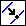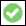> > > Split 2D geometry

Split 2D geometry
You can split elements into smaller segments. This can be useful for deleting and moving segments of profiles.
To split 2D geometry,
1. Click Modeling and then, in the Modify 2D group, click More.
2. ClickSplit 2D in the Tools section. The Split Edge dialog box opens.
3. Click or box the geometry element(s) to be split.
4. If you have specified one geometry element, specify the position on the element where you want it to be split, or select one of the following options and split the element as described:
Midpoint - Clicking this option causes a vertex to be generated at the midpoint of the element, creating two equal segments.
Segments - Type a positive number in the data entry field to specify how many segments of equal length should be created. Specifying two segments is equivalent to selecting the Midpoint option. (You can also enter non-integral numbers: The decimal produces a smaller segment proportional in length to one of the "whole" segments by that fractional value. For example, splitting an element into 2.5 segments produces two equidistant segments and one segment half their length.)
Length - Clicking this option causes the selected element to be labeled at its begin point (with 0.0) and at its end point (with its arc length). Enter a positive number in the data entry field to specify the split point (that is, the length of the first segment) measured from the begin point of the element. Enter a negative number to specify the split point measured from the end point of the element.
If you have specified more than one element, the elements will split each other.
5. Click OKto complete the operation.
If you want to split Splines with the midpoint or segments option, please keep in mind that the length calculation of a spline is an iterative and approximate process. In this case, the length of the edge to split is calculated with an absolute tolerance of 1e-2 to avoid excessive computation times for long splines.
After the length of the edge to split is calculated, the value obtained is divided into the necessary number of segments. The algorithm then searches the points on the edge that will define the arcs with the calculated lengths. As this length is already approximated and the length calculation used for finding the arc lengths again introduces an approximation error, the segments are not guaranteed to be of equal length.
The resulting error in terms of arc length of the segments can be estimated to less than (1e-2/no_segments + 1e-2).